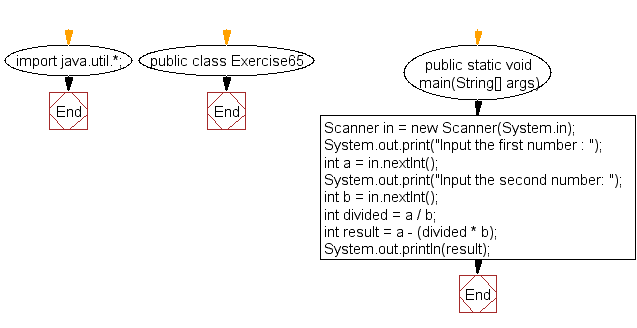﻿ Java: Calculate modules of 2 numbers without using modulus# Java Exercises: Calculate the modules of two numbers without using any inbuilt modulus operator

## Java Basic: Exercise-65 with Solution

Write a Java program to calculate the modules of two numbers without using any inbuilt modulus operator.
Test data:
Input the first number : 5
Input the second number: 3
2
Input the first number : 19
Input the second number: 7
5

Sample Solution:

Java Code:

``````import java.util.*;
public class Exercise65 {
public static void main(String[] args)
{
Scanner in = new Scanner(System.in);
System.out.print("Input the first number : ");
int a = in.nextInt();
System.out.print("Input the second number: ");
int b = in.nextInt();
int divided = a / b;
int result = a - (divided * b);
System.out.println(result);
}
}
```
```

Sample Output:

```Input the first number : 19
Input the second number: 7
5
```

Flowchart:Java Code Editor:

What is the difficulty level of this exercise?

Test your Programming skills with w3resource's quiz.

﻿

## Java: Tips of the Day

countOccurrences

Counts the occurrences of a value in an array.

Use Arrays.stream().filter().count() to count total number of values that equals the specified value.

```public static long countOccurrences(int[] numbers, int value) {
return Arrays.stream(numbers)
.filter(number -> number == value)
.count();
}
```

Ref: https://bit.ly/3kCAgLb